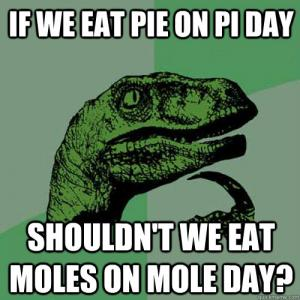### By the end of this unit students will be able to:

• Write a mole statement for an atom, molecule, or ionic compound.
• Define percent composition.
• Distinguish between an empirical and molecular formula of a compound.
• Calculate the formula mass of any ionic or covalent compound.
• Solve for percent composition for each element in any ionic or covalent compound.
• Determine the empirical and molecular formula of a compound.

Video: Calculating Formula Mass

Video: Calculating Molar Mass

Video: Mole Conversions

Video: Percent Composition

Video: How To Find The Empirical Formula of a Hydrated Ionic Compound

Video: Empirical Formula & Molecular Formula Determination From Percent Composition